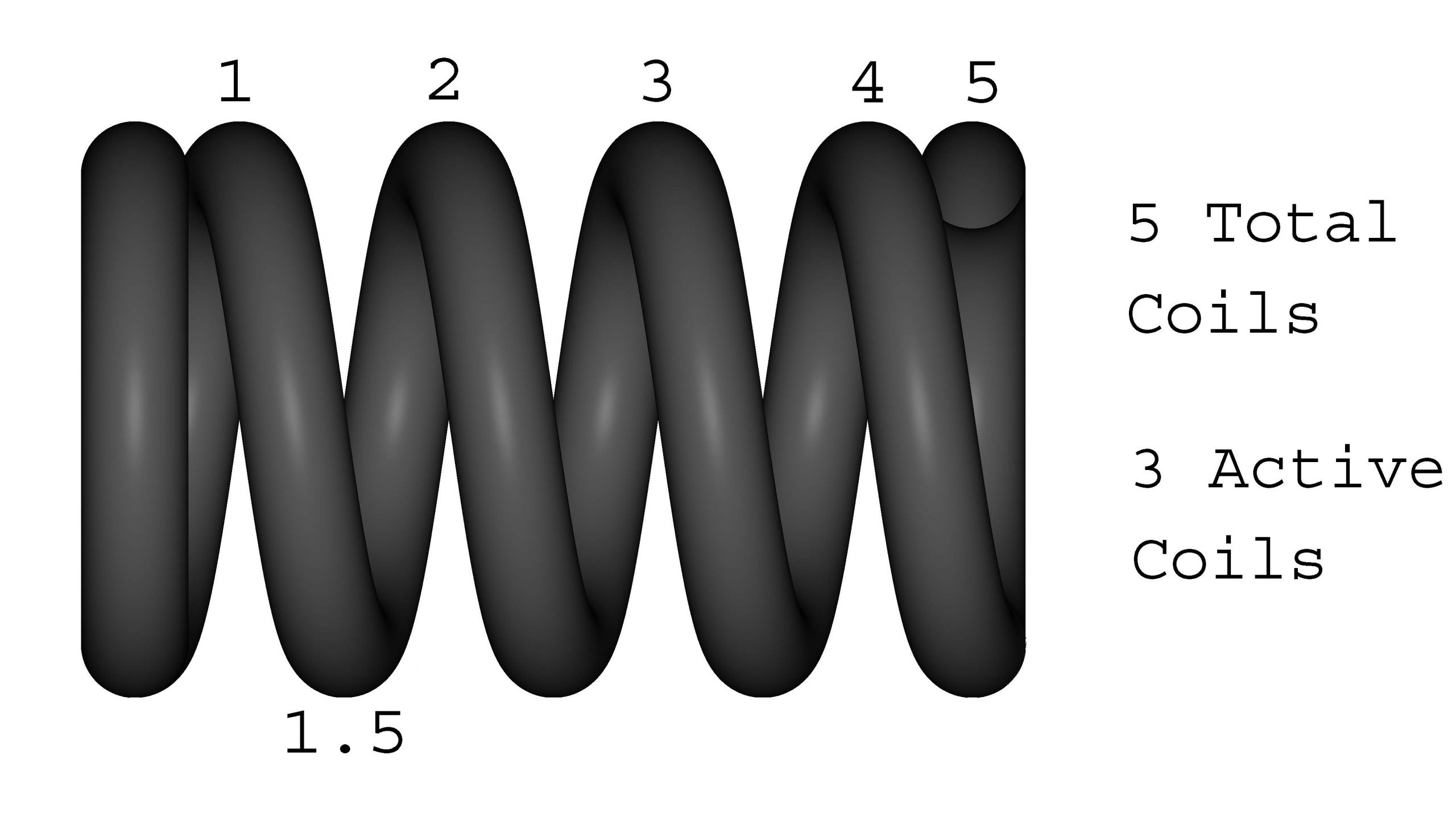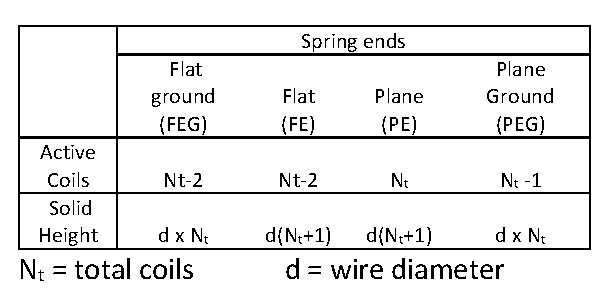# Design Tips: Coil CountIt may be simplest feature to measure, but the number of coils accounts for an astonishing number of errors in drawings and in new product development.  Counting them is easy enough – every rotation of 360 degrees equals one coil(90 degrees  = ¼ coil, 180 degrees = ½ coil).  However, the coil count can be critical and effects every functional aspect of a spring.

If a rate or load is specified coil count must be reference only

Spring rates, and by extension all spring forces, are controlled by 4 variables: coil count, spring diameter, wire diameter and material.  Under most circumstances the coil count is the only variable the spring manufacture has control over.  Tensile strength in wire can vary as much as 10%.  Because of this, two identical springs can have two different spring rates.  In order to hit a specified spring rate the manufacturer must be able to change the number of coils.  Putting a tolerance on both the spring rate and the coil count is spring maker’s equivalent of putting a square peg into a round hole.

Compression Springs and active coils

The number of coils on a compression spring are divided into two categories, active and inactive.  The active coils do all the work and handle all of the stresses.  Use the active coils when doing any calculations for stresses or loads.The inactive or dead coils to not support any of the load.  They are usually the close wound coils used to square up the ends of the spring.  Additional dead coils can usually be added to increase the solid height, to assemble a spring attachment or to decrease tangling.

Torsion Springs

Torsion springs also have an active coil count that may be different from the total coil count.  When the torsion legs are long and the moment is applied a significant distance from the centerline of the spring the wire in the torsion legs must also be considered as active coils.  Use the formula below to approximate the effect of the torsion legs on the active coils.

Active coils = Total coils + Leg Lengths / (Pi x Dmean)

Torsion springs present another challenge because the coil count also dictates the free position of the spring.  If you want the legs at 90 degrees, you need ¼ of a coil.  This limits the manufacturer’s options as far as controlling the torque and spring rate.  Under most circumstances it is not possible to put a tolerance on spring rates of torsion springs.

Extension Springs

The number of active coils on an extension spring is almost always equal to the total number of coils.  But, in addition to the spring rate, the number of coils directly effects the free length of an extension spring.  For normal loops the free length is defined by the following formula;

Free Length = (wire diameter x coil count) + (2 x inside diameter)

It is possible to cheat on this formula.  Shorter half loops can be formed to somewhat reduce the free length and extended hooks can be added to lengthen it.